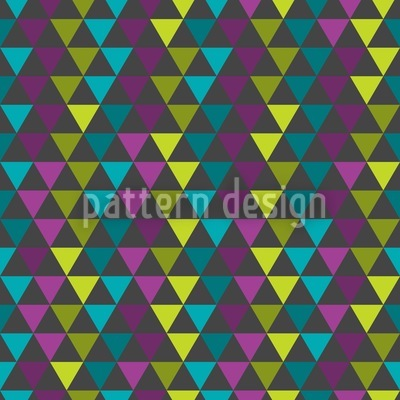••••# The Matrix Of The Triangles Seamless Vector Pattern Design

Keywords
The Matrix Of The Triangles design, The Matrix Of The Triangles vector, The Matrix Of The Triangles pattern, The Matrix Of The Triangles all over print, The Matrix Of The Triangles illustration, The Matrix Of The Triangles ornament, The Matrix Of The Triangles pattern design, The Matrix Of The Triangles artwork, The Matrix Of The Triangles vector art, The Matrix Of The Triangles background pattern, The Matrix Of The Triangles textile, The Matrix Of The Triangles seamless, The Matrix Of The Triangles repeating pattern, The Matrix Of The Triangles surface
Designer Christoph Stichlberger ID #4843
Description Columns of triangles with different light and color intensity.
Prices